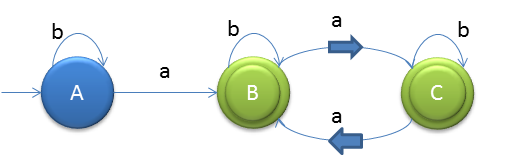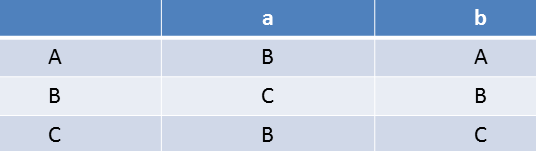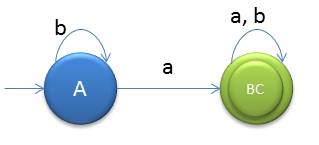# Minimization of DFA Example 1

Minimize the below DFA using partition method.First design its transition table### 0 equivalent

[A] and [B, C] (final and non-final)

### 1 equivalent

We will check for B's and C's outputs for input 'a' and 'b' but they are in the same set
As for B on input 'a' o/p is C and for C on input 'a' o/p is B, o/ps are present in same set.
And for B on input 'b' o/p is B and for C on input 'b' o/p is C again both are present in the same set.

So we stop here as there is no change in the sets. But if you see the states are minimized from 3 to 2.